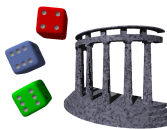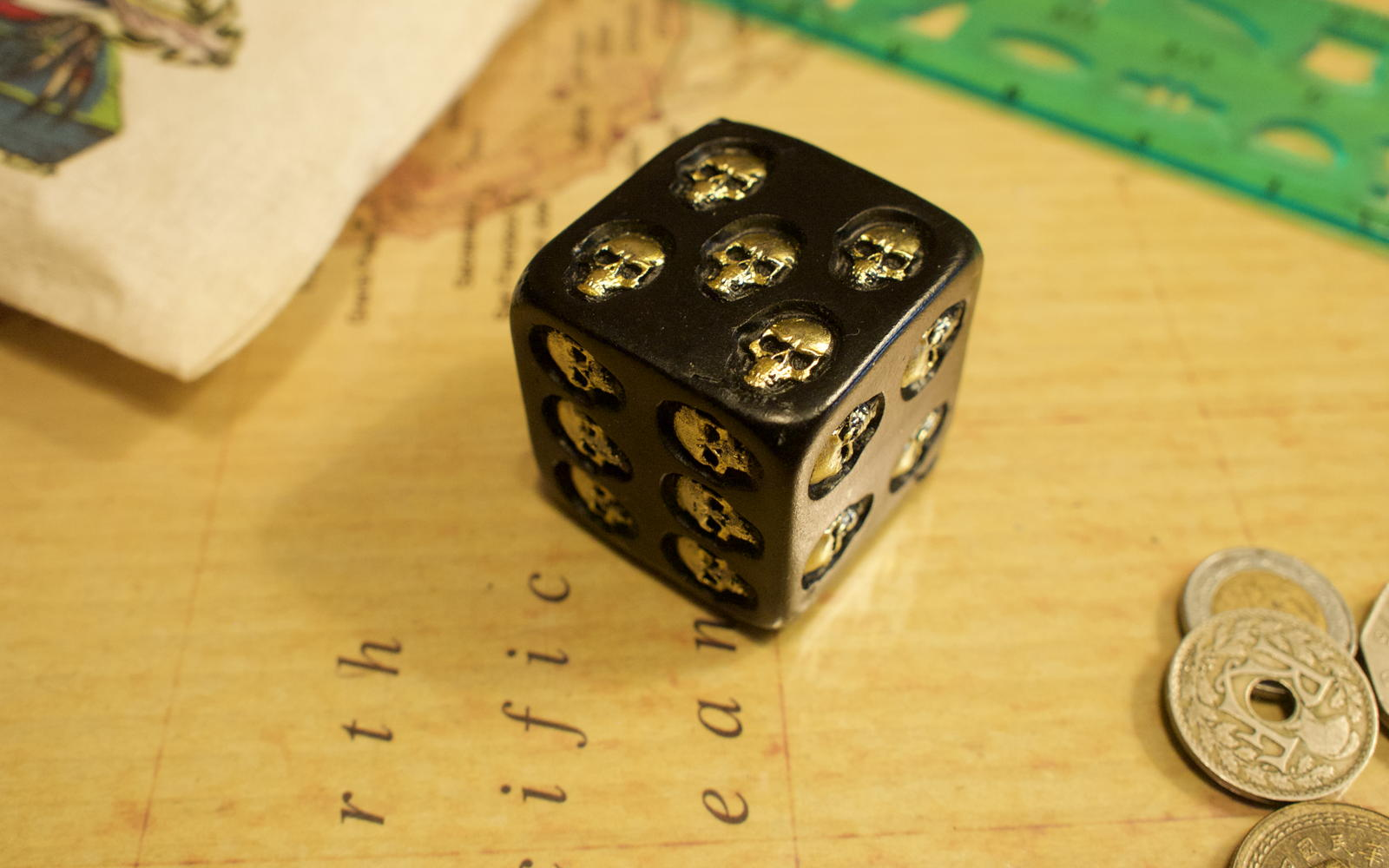# Biblyon the Great

This zine is dedicated to articles about the fantasy role-playing game Gods & Monsters, and other random musings.

Gods & Monsters Fantasy Role-Playing

Beyond here lie dragons

# Command-line Die SquareIs it any surprise that a die covered in skulls is biased?

Because Pythonista does not contain scipy, calculating chi-square values using it can have trouble on edge cases. This command-line script can run on data files created by the DieSquare mobile app, and uses scipy to make more reliable calculations.

Specify the DieSquare data file on the command line.

• \$ ~/bin/diesquare "Bronze d20.diesquare"
• Degrees of freedom: 19.0 X-square: 20.6
• p-value: 0.359317617197
• d20 bias is unlikely.

You can also specify the die size and a file of tab-delimited or colon-delimited data. The file should contain two columns: the result, and how many of those results occurred.

The DieSquare file format is:

• d6
• 1: 3
• 2: 16
• 3: 9
• 4: 8
• 5: 6
• 6: 18

That is, any line beginning with a lower-case “d” is assumed to be specifying the die size; any line with a number followed by a colon and space followed by a number is assumed to be a result. You can also put comments in by preceding the line with a pound symbol (#).

And as you might guess, this die is almost certainly biased.

• \$ ~/bin/diesquare "Skull d6.diesquare"
• Degrees of freedom: 5.0 X-square: 17.0
• p-value: 0.00449979697797
• d6 bias is probable.

The code itself (Zip file, 1.6 KB) is very simple.

[toggle code]

• #!/usr/bin/python
• #http://godsmonsters.com/Features/my-dice-random/diesquare-ios/command-line-die-square/
• import argparse
• import scipy.stats
• parser = argparse.ArgumentParser(description='Calculate Chi Square p-value using DieSquare data files.')
• args = parser.parse_args()
• class ChiSquare():
• def __init__(self, die, rolls):
• self.die = die
• self.parseRolls(rolls)
• def parseRolls(self, rolls):
• self.rollCount = 0
• self.rolls = {}
• for roll in rolls:
• if not roll:
• continue
• if roll.startswith('d'):
• self.die = int(roll[1:])
• continue
• if roll.startswith('#'):
• continue
• if "\t" in roll:
• separator = "\t"
• elif ": " in roll:
• separator = ": "
• else:
• print("Unknown separator in line ", roll)
• continue
• roll, count = (int(x) for x in roll.split(separator))
• self.rolls[roll] = count
• self.rollCount += count
• if args.verbose:
• print(self.rollCount)
• print(self.rolls)
• def calculate(self):
• if args.verbose:
• print '\n# ', self.die
• expected = float(self.rollCount)/float(self.die)
• freedom = float(self.die - 1)
• observed = self.rolls.values()
• expected = [expected]*self.die
• chisquare, pvalue = scipy.stats.chisquare(observed, expected)
• print "Degrees of freedom:", freedom, "X-square:", chisquare
• print "p-value:", pvalue
• if pvalue < 0 or pvalue > 1:
• color = '\033[5m\033[91m'
• result = 'unknown; data generates an invalid p-value'
• elif pvalue < .05:
• if pvalue < .01:
• color = '\033[91m'
• result = 'probable'
• else:
• color = '\033[33m'
• result = 'possible'
• else:
• result = 'unlikely'
• color = '\033[32m'
• #curses.start_color()
• print
• print color + 'd' + unicode(self.die) + ' bias is ' + result + '.\033[0m'
• calculator = ChiSquare(args.die, args.data)
• calculator.calculate()

Half of the code is just parsing the datafile; the actual calculation is a couple of lines using scipy:

• observed = self.rolls.values()
• expected = [expected]*self.die
• chisquare, pvalue = scipy.stats.chisquare(observed, expected)

The variable “observed” is the list of observed result counts. The variable “expected” is the list of expected result counts. For example, rolling a d6 60 times, the expected result count is 10 for each result, so expected will equal “[10, 10, 10, 10, 10, 10]”. And observed will be the actual results; for example, in the above data the die face 1 was rolled three times, 2 sixteen times, 3 nine times, 4 eight times, 5 six times, and 6 eighteen times. This is the list “[3, 16, 9, 8, 6, 18]”. The script, of course, uses the lists it constructed by reading the datafile.

In response to DieSquare for iOS: Are your dice biased? Perform on-the-fly chi-square tests using your iPad or iPhone and Pythonista.